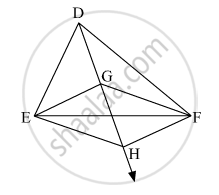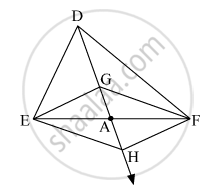Advertisement Remove all ads

# In the Given Figure, G is the Point of Concurrence of Medians of δ Def. Take Point H on Ray Dg Such that D-g-h and Dg = Gh, Then Prove that - Geometry

Sum

In the given figure, G is the point of concurrence of medians of Δ DEF. Take point H on ray DG such that D-G-H and DG = GH, then prove that square GEHF is a parallelogram.Advertisement Remove all ads

#### SolutionG is the point of concurrence of the medians of Δ DEF.

Let the point where the median divides EF into two equal parts be A.
Thus, EA = AF.                     .....(1)
we know that the point of concurrence of the medians, divides each median in the ratio 2 : 1.
So, let DG = 2x  and GA = x
Given that DG = GH
So, GA = AH = x
Thus, point A dividess EF and GH into two equal parts.

Hence , squareGEHF is a parallelogram as the diagonals EF and GH bisect each other.

Is there an error in this question or solution?
Advertisement Remove all ads

#### APPEARS IN

Balbharati Mathematics 2 Geometry 9th Standard Maharashtra State Board
Chapter 5 Quadrilaterals
Practice Set 5.2 | Q 3 | Page 67
Advertisement Remove all ads
Advertisement Remove all ads
Share
Notifications

View all notifications

Forgot password?